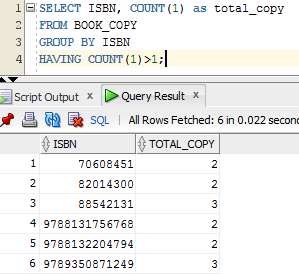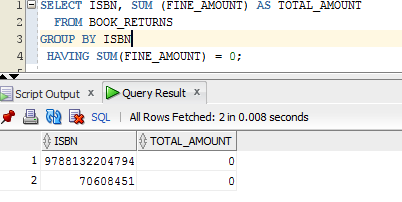# Having Clause in SQL

This is used along with GROUP BY clause to check the conditions on its function outputs like COUNT, SUM, MAX, MIN, AVG etc. we cannot directly use them in WHERE clause as these values are obtained after WHERE clause is evaluated. That means, the order executing any normal query is :

```SELECT columns		→ ?
WHERE Conditions	→ ?
GROUP BY columns	→ ?
HAVING conditions	→ ?
ORDER BY Columns; 	→ ?```

Hence ‘WHERE’ clause will not get those column values to filter the records. Hence we need a separate clause to check the values of group by condition. Thus HAVING clause is used.

```SELECT ISBN, COUNT (1) as total_copy
FROM BOOK_COPY
GROUP BY ISBN
HAVING COUNT (1)>1;```We can use any group by functions in the HAVING clause with operators like =, >, >=, <, <=, <>, !=, LIKE, NOT LIKE, IN, NOT IN, IS NULL, IS NOT NULL etc.

```SELECT ISBN, SUM (FINE_AMOUNT) AS TOTAL_AMOUNT
FROM BOOK_RETURNS
GROUP BY ISBN
HAVING SUM (FINE_AMOUNT) = 0;```Translate »